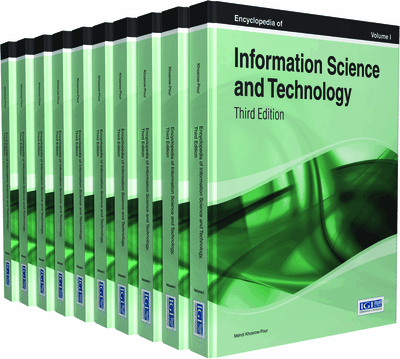Classification Reasoning as a Basic Part of Machine Learning

Xenia Naidenova (Military Medical Academy, Russia)
DOI: 10.4018/978-1-4666-5888-2.ch012
Available
\$37.50
No Current Special Offers

Top

Introduction

We focus on the logical or symbolic supervised methods of machine learning. This mode of learning covers mining logical rules and dependencies from data: “if-then” rules, decision trees, functional, and associative dependencies. This learning is also used for extracting concept from data sets, constructing rough sets, hierarchical classification of objects, mining ontology from data, generating hypotheses, and some others (Kotsiantis, 2007). It has been proven in (Naidenova, 1996) that the tasks of mining all logical dependencies from data sets are reduced to approximation of a given classification (partitioning) on a given set of object descriptions.

The search for best approximation of a given object classification leads to a concept of good classification (diagnostic) test firstly introduced in (Naidenova & Polegaeva, 1986). A good classification test has a dual nature. On the one hand, it makes up a logical expression in the form of implication, associative or functional dependency. On the other hand, it generates the partition of a training set of objects equivalent to a given classification (partitioning) of this set or the partition that is nearest to the given classification with respect to the inclusion relation between partitions (Cosmadakis et al., 1986).

We consider two ways for giving classifications as it is shown in Figure 1: (1) by a target attribute KL or (2) by a value v of target attribute. The target attribute partitions a given set of examples into disjoint classes the number of which is equal to the number of values of this attribute. The target value of attribute partitions a given set of examples into two disjoint classes: the examples in description of which the target value appears (positive examples); all the other examples (negative examples).

We are interested in solving the following tasks:

• 1.

Given attribute KL, to infer logical rules of the form:A B CKL orD SKL ororA S Q VKL

where A, B, C, D, Q, S, V – the names of attributes.

• 2.

Given value v of attribute KL, to infer logical rules of the form:if ((value of attribute А = “а”) &(value of attribute В = “b”) &………………………………………),then (value of attribute KL = “v”).

Rules of the first form are functional dependencies as they are determined in relational data base constructing. Rules of the second form are implicative dependencies as they are determined in association rule mining (Agarval et al., 2011). Left parts of rules can be considered as descriptions of given classifications or classes of objects. In our diagnostic test approach (the DTA) to logical rules mining, left parts of these rules constitute diagnostic tests.

Implicative assertions (logical rules of the first kind in our terminology) describe regular relationships connecting together objects, properties and classes of objects. The DTA enables one to mine a whole class of implicative assertions including not only simple implication (a, b, cd), but also forbidden assertion (a, b, cfalse (never), diagnostic assertion (x, da; x, b → not a; d, bfalse), assertion of alternatives (a or btrue (always); a, bfalse), compatibility (a, b, cVA, where VA is the occurrence’s frequency of rule).

Key Terms in this Chapter

Good Maximally Redundant Classification Test: (GMRT): A good test is a maximally redundant one if extending it by any attribute’s value not belonging to it changes its property “to be a good test” into the property “to be a test but not a good one.”

Diagnostic or Classification Test: Assume that we have two sets of objects’ examples called positive and negative examples, respectively. A test for a subset of positive examples is a set of common attributes’ values describing this subset, i.e. it is common or general feature for all examples of this subset and, simultaneously, none of the negative examples is described by it.

Good Irredundant Classification Test: (GIRT): A good test is irredundant if deleting any attribute’s value from it changes its property “to be test” into the property “not to be a test.”

Good Classification Test: A classification test describing a given set of positive examples is good if adding to it any positive example not belonging to it, implies that the set of attributes’ values describing the obtained set will describe at least one negative example.

Commonsense Reasoning Rules: (CRRs): These are rules with the help of which implicative assertions are used, updated and inferred from examples. The deductive CRRs are based on the use of syllogisms: modus ponens , modus ponendo tollens , modus tollendo ponens , and modus tollens . The inductive CRRs are the canons formulated by J. S. Mill (1872) .

Implicative Assertions: Implicative assertions describe regular relationships between objects, their properties, classes of objects, and between properties of different objects. They are represented in the “if-then” form and can be given explicitly by an expert or derived automatically from examples with the help of a learning process.

Inductive Transitions: These are the processes of extending or narrowing sets of values (objects). They can be smooth and boundary. Upon smooth transition, a certain assigned property of the generated sets does not change. Upon boundary transition, a certain assigned property of the generated sets changes to the opposite one.

Complete Chapter List

Search this Book:
Reset